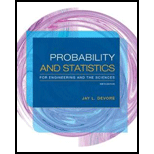# There are two traffic lights on a commuter’s route to and from work. Let X 1 be the number of lights at which the commuter must stop on his way to work, and X 2 be the number of lights at which he must stop when returning from work. Suppose these two variables are independent, each with pmf given in the accompanying table (so X 1 , X 2 is a random sample of size n = 2). x 1 0 1 2 p ( x 1 ) 0.2 0.5 0.3 µ =1.1, σ 2 =0.49 a. Determine the pmf of T o = X 1 + X 2 . b. Calculate µT o . How does it relate to µ , the population mean? c. Calculate σ 2 T o . How does it relate to σ 2 , the population variance? d. Let X 3 and X 4 be the number of lights at which a stop is required when driving to and from work on a second day assumed independent of the first day. With T o = the sum of all four X i ’s, what now are the values of E ( T o ) and V ( T o )? e. Referring back to (d), what are the values of P ( T 0 = 8) and P ( T o ≥ 7) [ Hint : Don’t even think of listing all possible outcomes!]### Probability and Statistics for Eng...

9th Edition
Jay L. Devore
Publisher: Cengage Learning
ISBN: 9781305251809### Probability and Statistics for Eng...

9th Edition
Jay L. Devore
Publisher: Cengage Learning
ISBN: 9781305251809

#### Solutions

Chapter
Section
Chapter 5.3, Problem 38E
Textbook Problem

## Expert Solution

### Want to see the full answer?

Check out a sample textbook solution.See solution

### Want to see this answer and more?

Experts are waiting 24/7 to provide step-by-step solutions in as fast as 30 minutes!*

See Solution

*Response times vary by subject and question complexity. Median response time is 34 minutes and may be longer for new subjects.